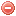# Description

The Keetch-Byram drought index ($$KBDI$$) was developed in the USA by Keetch and Byram (1968) in order to measure drought and facilitate fire control operations.

This index is cumulative and requires daily temperature, daily and annual precipitation as input data. It is aimed to reflect the dryness - and thus the flammability - of organic material in the ground by taking into account the effects of rainfall and evapotranspiration on moisture deficiency in deep duff and upper soil layers (Keetch & Byram 1968).

The $$KBDI$$ has been used for practical application in the Southeastern United States and to some extent in the Northeastern US (Burgan 1988). It has also been used for research studies in other regions, for instance the Hawaiian Islands and Northern Eurasia (Dolling et al. 2005, Groisman et al. 2007).

The $$KBDI$$ has been included in the US National Fire Danger Rating System (NFDRS; Burgan 1988, Melton 1989).

# Formula

The $$KBDI$$ is calculated as follows:

$\mathit{KBDI_t}=Q+\dfrac{(800-Q)\cdot{(0.968\cdot{e^{0.0486\cdot{T}}}-8.30)}\cdot{\Delta}t}{1+10.88\cdot{e^{-0.0441\cdot{P}}}}\cdot{10^{-3}}$

where $$Q$$ is the previous' day $$KBDI$$ minus net rainfall [in/100] (cf. below), $$T$$ air temperature [°F], $$\Delta t$$ time increment (one day) and $$P$$ mean annual precipitation [in].
For temperature the maximum air temperature (or the dry-bulb temperature at time of basic observation) should be used.

$Q = KBDI_{t-1} - {P_{net_t}}\cdot{ 100}$

$P_{net_t} = max \bigg[0 , P_t - max (0, P_{lim} - \displaystyle\sum _{i=1} ^{rr-1}P_{t-i})\bigg]$

with $$rr$$ as ther number of consecutive days with rain.

In order to obtain net rainfall, 0.2 inch has to be subtracted from any daily rainfall amount exceeding 0.2 inch. In the case of consecutive days with rainfall, the 0.2 inch has to be subtracted on the exact day when the summed rainfall amount exceeds 0.2 inch. If the daily rainfall amount is smaller than 0.2, then net rainfall equals zero. Finally, net rainfall, expressed in hundredth of inch, has to be subtracted from $$Q$$.

The $$KBDI$$ is cumulative, i.e. it is imperative to start the index computation when upper soil layer are saturated with water, e.g. after a period of abundant rainfall, such as 6 or 8 inches in a period of a week. The first index value is then set to 0.

NB: There is a typographical error in the original paper of Keetch and Byram (1968). The last constant in the numerator of the $$KBDI$$ equation should have been 8.3 (as indicated here) and not 0.830 (as indicated in the original publication; cf. Crane 1982 and Alexander 1990).

The $$KBDI$$ is supposed to be calculated on a daily basis. No specific time for the recording of meteorological data is stipulated. Thus the meteorological data used for its calculation are the meteorological data at the time of the basic weather observation.

A function of the $$KBDI$$ in [mm] has to be included in the $$FFDI$$ and the Sharples index formulas. The $$KBDI$$ in S.I. units with air temperature and precipitation expressed in [°C], and [mm] respectively, is calculated as follows (cf. Crane 1982 in Alexander 1990):

$\mathit{KBDI_{SI_t}}=Q_{SI}+\dfrac{(203.2-Q_{SI})\cdot{(0.968\cdot{e^{0.0875\cdot{T}+1.5552}}-8.30)}\cdot{\Delta}t}{1+10.88\cdot{e^{-0.001736\cdot{P}}}}\cdot{10^{-3}}$

with

$Q_{SI} = KBDI_{SI_{t-1}} - P_{net_t}$

It is also imperative to start the index computation when upper soil layer are saturated with water, e.g. after a period of abundant rainfall, such as 152 or 203 mm in a period of a week. The first index value is then set to 0.

# Index interpretation

The $$KBDI$$ expresses moisture deficiency in hundredths of an inch, and is based on a measurement of 8 inches (0.2 m) of available moisture in the upper soil layers that can be used by vegetation for evapotranspiration. Therefore, it is on a scale ranging from 0 to 800, zero being the point of no moisture deficiency and 800 the maximum possible drought.

The range of the $$KBDI$$ output values can be divided into several stages. However, although these values have a definite signification in terms of moisture deficiency, the meaning of a particular stage for fire activity and control must be determined locally (Keetch & Byram 1968). For an example of the determination of the relationships between the $$KBDI$$ and fuel flammability/fire activity, see Melton (1989).

Original publication:
Keetch & Byram (1968)

Other publications:
Burgan (1988)
Melton (1989)
Alexander (1990)
Dolling et al. (2005)
Groisman et al. (2007)

### Bibliography SearchNo results!

No results were found. Are you sure you searched for a tag?

### Symbols

 Variable Description Unit $$T$$ air temperature °C $$T_{dew}$$ dew point temperature °C $$H$$ air humidity % $$P$$ rainfall mm $$U$$ windspeed m/s $$w$$ days since last rain (or rain above threshold) d $$rr$$ days with consecutive rain d $$\Delta t$$ time increment d $$\Delta{e}$$ vapor pressure deficit kPa $$e_s$$ saturation vapor pressure kPa $$e_a$$ actual vapor pressure kPa $$p_{atm}$$ atmospheric pressure kPa $$PET$$ potential evapotranspiration mm/d $$r$$ soil water reserve mm $$r_s$$ surface water reserve mm $$EMC$$ equilibrium moisture content % $$DF$$ drought factor - $$N$$ daylight hours hr $$D$$ weighted 24-hr average moisture condition hr $$\omega$$ sunset hour angle rad $$\delta$$ solar declination rad $$\varphi$$ latitude rad $$Cc$$ cloud cover Okta $$J$$ day of the year (1..365/366) - $$I$$ heat index - $$R_n$$ net radiation MJ⋅m-2⋅d-1 $$R_a$$ daily extraterrestrial radiation MJ⋅m-2⋅d-1 $$R_s$$ solar radiation MJ⋅m-2⋅d-1 $$R_{so}$$ clear-sky solar radiation MJ⋅m-2⋅d-1 $$R_{ns}$$ net shortwave radiation MJ⋅m-2⋅d-1 $$R_{nl}$$ net longwave radiation MJ⋅m-2⋅d-1 $$\lambda$$ latent heat of vaporization MJ/kg $$z$$ elevation m a.s.l. $$d_r$$ inverse relative distance Earth-Sun - $$\alpha$$ albedo or canopy reflection coefficient - $$\Delta$$ slope of the saturation vapor pressure curve kPa/°C $$Cc$$ cloud cover eights $$ROS$$ rate of spread m/h $$RSF$$ rate of spread factor - $$WF$$ wind factor - $$WRF$$ water reserve factor - $$FH$$ false relative humidity - $$FAF$$ fuel availability factor - $$PC$$ phenological coefficient -

 Suffix Description $$-$$ mean / daily value $$_{max}$$ maximum value $$_{min}$$ minimum value $$_{12}$$ value at 12:00 $$_{13}$$ value at 13:00 $$_{15}$$ value at 15:00 $$_{m}$$ montly value $$_{y}$$ yearly value $$_{f/a}$$ value at fuel-atmosphere interface $$_{dur}$$ duration $$_{soil}$$ value at soil level

 Constant Description $$e$$ Euler's number $$\gamma$$ psychrometric constant $$G_{SC}$$ solar constant $$\sigma$$ Stefan-Bolzmann constant